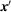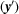International Tables for Crystallography (2015). Vol. A, ch. 1.5, pp. 75-105https://doi.org/10.1107/97809553602060000923

## Contents

• 1.5. Transformations of coordinate systems  (pp. 75-106)
• 1.5.1. Origin shift and change of the basis  (pp. 75-83) | html | pdf |
• 1.5.1.1. Origin shift  (pp. 75-76) | html | pdf |
• 1.5.1.2. Change of the basis  (pp. 76-83) | html | pdf |
• 1.5.1.3. General change of coordinate system  (p. 83) | html | pdf |
• 1.5.2. Transformations of crystallographic quantities under coordinate transformations  (pp. 83-87) | html | pdf |
• 1.5.2.1. Covariant and contravariant quantities  (p. 83) | html | pdf |
• 1.5.2.2. Metric tensors of direct and reciprocal lattices  (p. 84) | html | pdf |
• 1.5.2.3. Transformation of matrix–column pairs of symmetry operations  (p. 84) | html | pdf |
• 1.5.2.4. Augmented-matrix formalism  (pp. 84-86) | html | pdf |
• 1.5.2.5. Example: paraelectric-to-ferroelectric phase transition of GeTe  (pp. 86-87) | html | pdf |
• 1.5.3. Transformations between different space-group descriptions  (pp. 87-90) | html | pdf |
• 1.5.3.1. Space groups with more than one description in this volume  (pp. 87-88) | html | pdf |
• 1.5.3.2. Examples  (pp. 88-90) | html | pdf |
• 1.5.3.2.1. Transformations between different settings of P21/c  (pp. 88-90) | html | pdf |
• 1.5.3.2.2. Transformation between the two origin-choice settings of I41/amd  (p. 90) | html | pdf |
• 1.5.4. Synoptic tables of plane and space groups  (pp. 91-106) | html | pdf |
• 1.5.4.1. Additional symmetry operations and symmetry elements  (pp. 91-95) | html | pdf |
• 1.5.4.1.1. Determining the type of a symmetry operation  (pp. 91-92) | html | pdf |
• 1.5.4.1.2. Cosets without additional types of symmetry elements  (p. 92) | html | pdf |
• 1.5.4.1.3. Examples with additional types of symmetry elements  (pp. 92-95) | html | pdf |
• 1.5.4.2. Synoptic table of the plane groups  (p. 95) | html | pdf |
• 1.5.4.3. Synoptic table of the space groups  (pp. 95-106) | html | pdf |
• References | html | pdf |
• Figures
• Fig. 1.5.1.1. The coordinates of the points X (or Y) with respect to the old origin O are x (y), and with respect to the new originthey are(p. 75) | html | pdf |
• Fig. 1.5.1.2. Monoclinic centred lattice, projected along the unique axis  (p. 80) | html | pdf |
• Fig. 1.5.1.3. Body-centred cell I with aI, bI, cI and a corresponding primitive cell P with aP, bP, cP  (p. 80) | html | pdf |
• Fig. 1.5.1.4. Face-centred cell F with aF, bF, cF and a corresponding primitive cell P with aP, bP, cP  (p. 80) | html | pdf |
• Fig. 1.5.1.5. Tetragonal lattices, projected along(p. 81) | html | pdf |
• Fig. 1.5.1.6. Unit cells in the rhombohedral lattice  (p. 81) | html | pdf |
• Fig. 1.5.1.7. Hexagonal lattice projected along(p. 82) | html | pdf |
• Fig. 1.5.1.8. Hexagonal lattice projected along(p. 82) | html | pdf |
• Fig. 1.5.1.9. Rhombohedral lattice with a triple hexagonal unit cell a, b, c in obverse setting  (p. 82) | html | pdf |
• Fig. 1.5.1.10. Rhombohedral lattice with primitive rhombohedral cell arh, brh, crh and the three centred monoclinic cells  (p. 82) | html | pdf |
• Fig. 1.5.2.1. Illustration of the transformation of symmetry operations, also called a `mapping of mappings'  (p. 84) | html | pdf |
• Fig. 1.5.3.1. Three possible cell choices for the monoclinic space group(14) with unique axis b  (p. 87) | html | pdf |
• Fig. 1.5.3.2. Two possible origin choices for the orthorhombic space group Pban (50)  (p. 88) | html | pdf |
• Fig. 1.5.3.3. General-position diagram of the space group R3m (160) showing the relation between the hexagonal and rhombohedral axes in the obverse setting  (p. 88) | html | pdf |
• Fig. 1.5.4.1. Symmetry-element diagram for space group P23 (195)  (p. 95) | html | pdf |
• Tables
• Table 1.5.1.1. Selected 3 × 3 transformation matricesand(pp. 77-80) | html | pdf |
• Table 1.5.3.1. Transformation of reflection-condition data for P121/c1 to P1121/a  (p. 89) | html | pdf |
• Table 1.5.4.1. Additional symmetry operations and their locations if the translation vector t is inclined to the symmetry axis or symmetry plane  (p. 93) | html | pdf |
• Table 1.5.4.2. Additional symmetry operations due to a centring vector t and their locations  (p. 94) | html | pdf |
• Table 1.5.4.3. List of plane-group symbols  (p. 96) | html | pdf |
• Table 1.5.4.4. List of space-group symbols for various settings and cells  (pp. 97-105) | html | pdf |